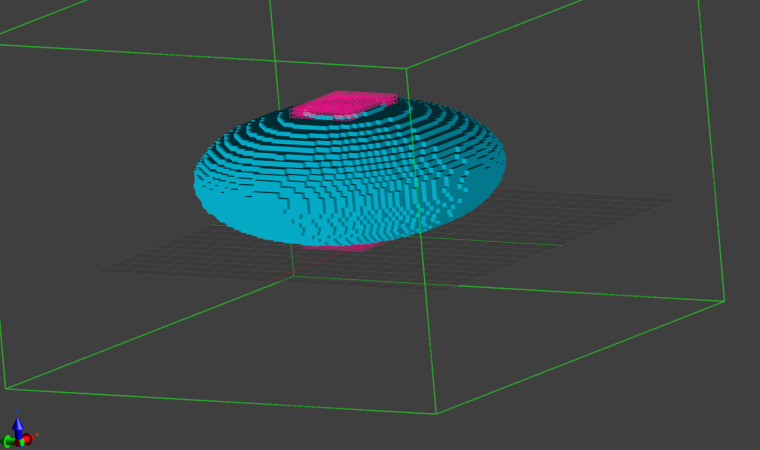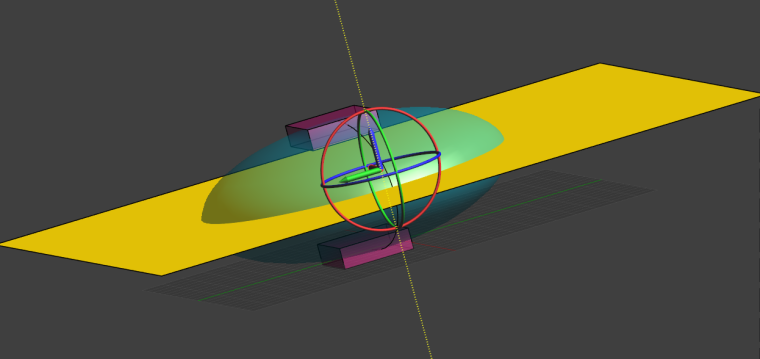# Compute the total current flowing between two electrodes in an Electro Quasistatic LF simulation

Assume we have an LF simulation with two electrodes, at + and - 1V, respectively, as shown in the voxel view below. We want to compute the total current flowing between the two electrodes.We start by creating a plane that cut through the computational domain, with each electrode in either part of the plane:We then use the Flux Evaluator to compute the total current flowing through the plane:

Is there a disadvantage to calculating the current by using the power loss in the simulation domain and avoiding extra geometry? (i.e. R = (V^2)/P and then I = V/R using the "Total Loss" in "All Regions" from the SAR statistics evaluator?)

The "Total Loss" approach works too. Be aware that it assumes that all the power dissipated by the system occurs via the Joule effect in conductive materials. In other words, it only accounts for Ohmic currents and neglects the contribution of Displacement current (like the current that flows between the two plates separated by air in a capacitor).
This means you can use this approach if you could have used the Ohmic Quasi-Static solver instead of the Quasi-Static one (since the former neglects displacement currents, while the latter does not).
For currents flowing between electrodes placed on a human body, displacement currents are typically negligible.